# Posts tagged as “range”

Problem:

Implement a MyCalendarTwo class to store your events. A new event can be added if adding the event will not cause a triple booking.

Your class will have one method, book(int start, int end). Formally, this represents a booking on the half open interval [start, end), the range of real numbers x such that start <= x < end.

triple booking happens when three events have some non-empty intersection (ie., there is some time that is common to all 3 events.)

For each call to the method MyCalendar.book, return true if the event can be added to the calendar successfully without causing a triple booking. Otherwise, return false and do not add the event to the calendar.

Your class will be called like this: MyCalendar cal = new MyCalendar(); MyCalendar.book(start, end)

Example 1:

Note:

• The number of calls to MyCalendar.book per test case will be at most 1000.
• In calls to MyCalendar.book(start, end)start and end are integers in the range [0, 10^9].

Idea:

Brute Force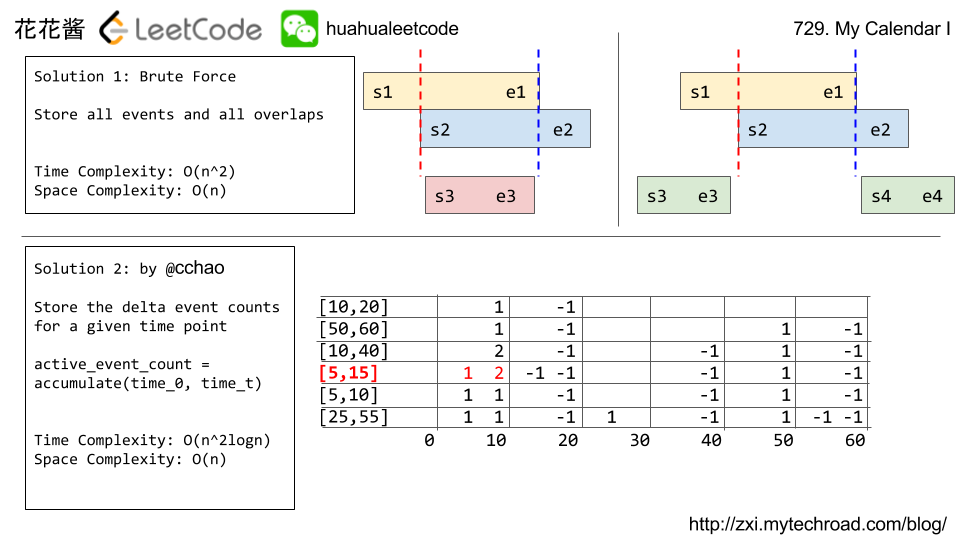Solution1:

Brute Force

Time Complexity: O(n^2)

Space Complexity: O(n)

Java

Python

Solution 2:

Counting

Time Complexity: O(n^2logn)

Space Complexity: O(n)

Related Problems:

Problem:

Implement a MyCalendar class to store your events. A new event can be added if adding the event will not cause a double booking.

Your class will have the method, book(int start, int end). Formally, this represents a booking on the half open interval [start, end), the range of real numbers x such that start <= x < end.

double booking happens when two events have some non-empty intersection (ie., there is some time that is common to both events.)

For each call to the method MyCalendar.book, return true if the event can be added to the calendar successfully without causing a double booking. Otherwise, return false and do not add the event to the calendar.

Your class will be called like this: MyCalendar cal = new MyCalendar(); MyCalendar.book(start, end)

Example 1:

Note:

• The number of calls to MyCalendar.book per test case will be at most 1000.
• In calls to MyCalendar.book(start, end)start and end are integers in the range [0, 10^9].

Idea:

Binary Search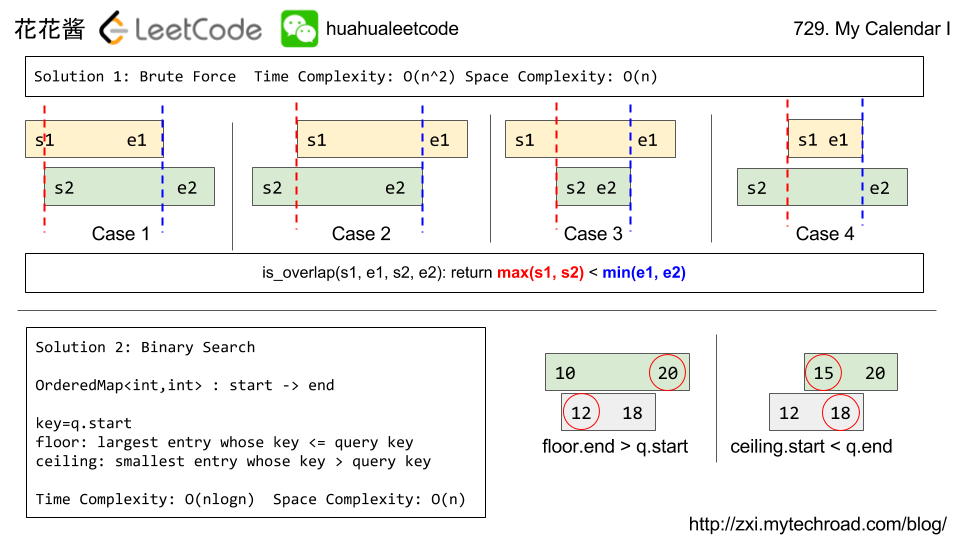Solution1:

Brute Force: O(n^2)

C++

Solution 2:

Binary Search O(nlogn)

C++

Java

Related Problems:

Problem:

On an infinite number line (x-axis), we drop given squares in the order they are given.

The i-th square dropped (positions[i] = (left, side_length)) is a square with the left-most point being positions[i] and sidelength positions[i].

The square is dropped with the bottom edge parallel to the number line, and from a higher height than all currently landed squares. We wait for each square to stick before dropping the next.

The squares are infinitely sticky on their bottom edge, and will remain fixed to any positive length surface they touch (either the number line or another square). Squares dropped adjacent to each other will not stick together prematurely.

Return a list ans of heights. Each height ans[i] represents the current highest height of any square we have dropped, after dropping squares represented by positions, positions, ..., positions[i].

Example 1:

Example 2:

Note:

• 1 <= positions.length <= 1000.
• 1 <= positions[i] <= 10^8.
• 1 <= positions[i] <= 10^6.

Idea:

Range query with map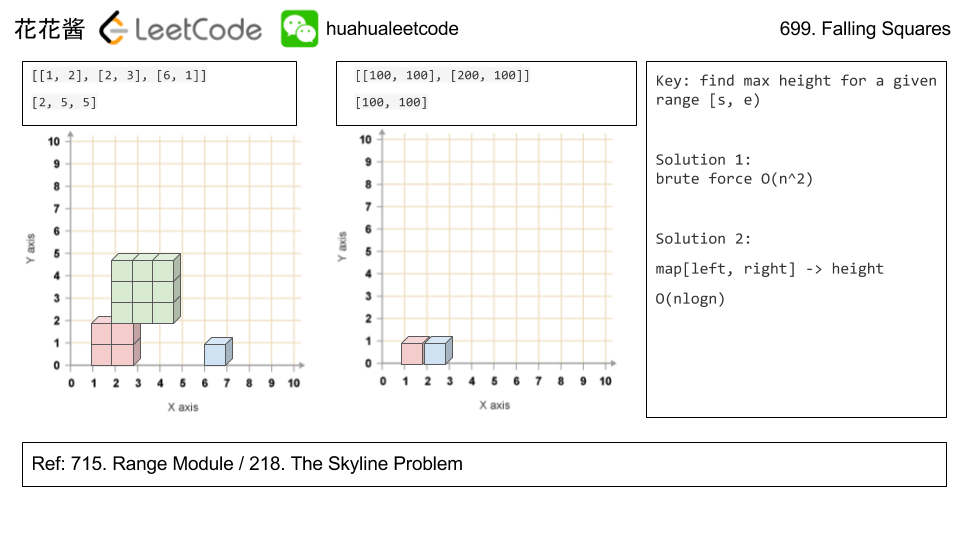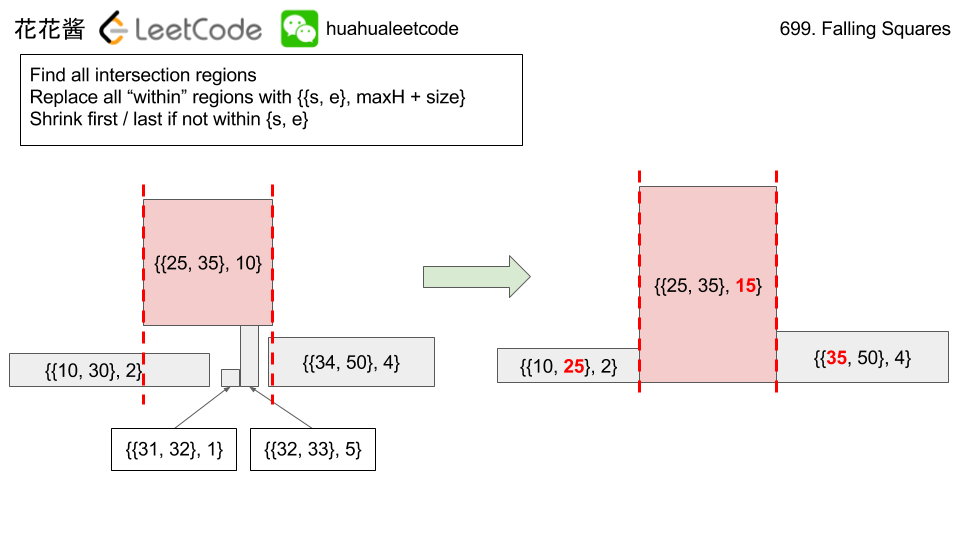Solution:

C++ map

C++ vector without merge

C++ / vector with merge (slower)

Related Problems:

Problem:

A Range Module is a module that tracks ranges of numbers. Your task is to design and implement the following interfaces in an efficient manner.

• addRange(int left, int right) Adds the half-open interval [left, right), tracking every real number in that interval. Adding an interval that partially overlaps with currently tracked numbers should add any numbers in the interval [left, right) that are not already tracked.
• queryRange(int left, int right) Returns true if and only if every real number in the interval [left, right) is currently being tracked.
• removeRange(int left, int right) Stops tracking every real number currently being tracked in the interval [left, right).

Example 1:

Note:

• A half open interval [left, right) denotes all real numbers left <= x < right.
• 0 < left < right < 10^9 in all calls to addRange, queryRange, removeRange.
• The total number of calls to addRange in a single test case is at most 1000.
• The total number of calls to queryRange in a single test case is at most 5000.
• The total number of calls to removeRange in a single test case is at most 1000.

Idea:

map / ordered ranges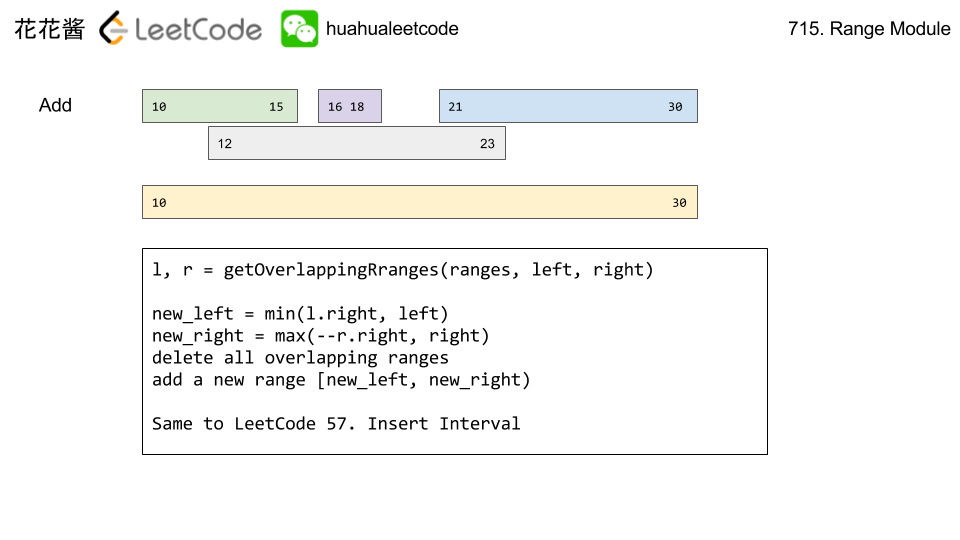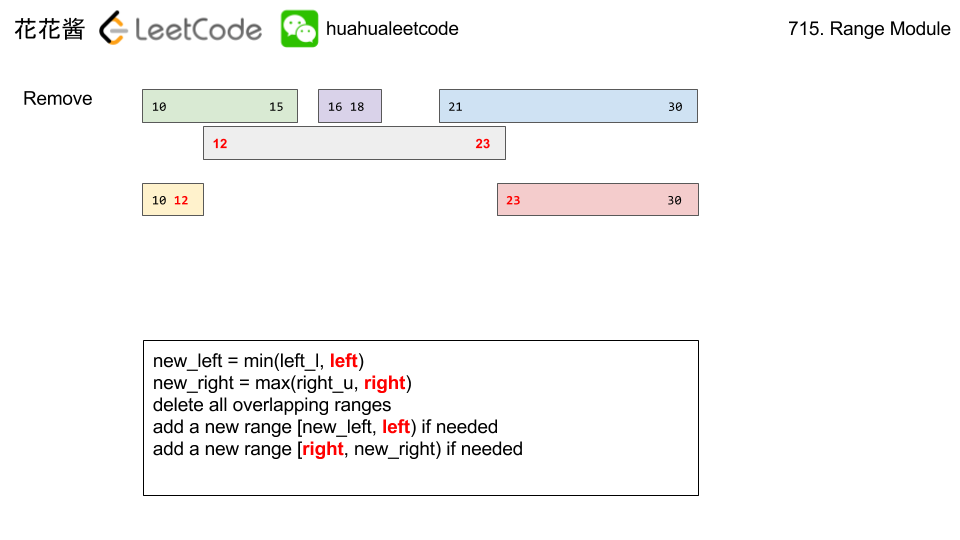Solution:

C++ / vector

C++ / map

Related Problems:

Mission News Theme by Compete Themes.